# Symplectic space

(diff) ← Older revision | Latest revision (diff) | Newer revision → (diff)

An odd-dimensional projective space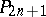over a fieldendowed with an involutory relation which is a null polarity; it is denoted by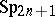.

Let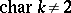. The absolute null polarity incan always be written in the form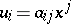, where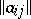is a skew-symmetric matrix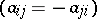. In vector form, the absolute null polarity can be written in the form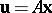, whereis a skew-symmetric operator whose matrix, in a suitable basis, reduces to the form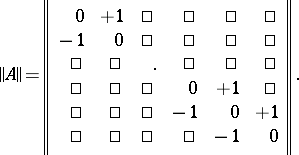In this case the absolute null polarity takes the canonical formThe absolute null polarity induces a bilinear form, written in canonical form as follows: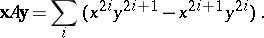Collineations ofthat commute with its null polarity are called symplectic transformations; the operators defining these collineations are called symplectic. The above canonical form ofdefines the square matrix of order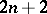of a symplectic operatorwhose elements satisfy the conditionswhere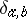is the Kronecker delta. Such a matrix is called symplectic; its determinant is equal to one. The symplectic transformations form a group, which is a Lie group.

Every point of the spacelies in its polar hyperplane with respect to the absolute null polarity. One can also define polar subspaces in. The manifold of self-polar-spaces ofis called its absolute linear complex. In this context, a symplectic group is also called a (linear) complex group.

Every pair of straight lines, and their polar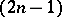-spaces in the null polarity, define a unique symplectic invariant inwith respect to the group of symplectic transformations of this space. Through every point of each line there passes a transversal of this line and-spaces, which defines a projective quadruple of points. This is the geometrical interpretation of a symplectic invariant, which asserts the equality of the cross ratios of these quadruples of points.

The symplectic-dimensional space admits an interpretation in hyperbolic space and this indicates, among other things, a connection between symplectic and hyperbolic spaces. Thus, the group of symplectic transformations ofis isomorphic to the group of motions of the hyperbolic space. In this interpretation, the symplectic invariant is related to the distance between points in hyperbolic space.

How to Cite This Entry:
Symplectic space. Encyclopedia of Mathematics. URL: http://encyclopediaofmath.org/index.php?title=Symplectic_space&oldid=14721
This article was adapted from an original article by L.A. Sidorov (originator), which appeared in Encyclopedia of Mathematics - ISBN 1402006098. See original article